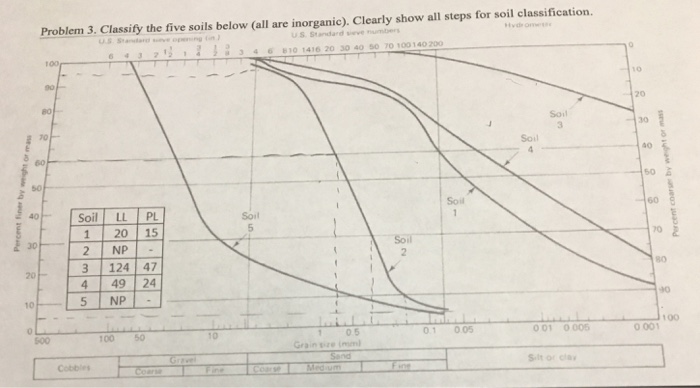Homework Help Question & Answers

Problem 3. Classify the five soils below (all are inorganic). Clearly show all steps for soil cla...Problem 3. Classify the five soils below (all are inorganic). Clearly show all steps for soil classification. US Standard seve numbers , 6 810141620 30405070 00140200 3 6 100 10 20 Soil 30 70 Soil 40 so a 50 60 40Soil LL PL Soil 120 15 2 NP 3 124 47 4 49 24 5 NP- 70 80 #0 10 001 0005 01 005 105 Grain size imm

Solution –

Atterberg’s limit are constrained to fine grained soil only, that’s why the soils with no fine grained content (soil 2 and 5) have no liquid limit and plastic limit.

1. Soil 1 –

Soil have both sand and silt or clay in it, bit major proportion of silt/clay is there so it is primarily a fine-grained soil.

To distinguish between silt or clay we will use A-line equation.

Liquid limit (LL) = 20 %

Plastic Limit (PL) = 15 %

Plasticity index (IP) = LL – PL = 20 – 15 = 5

A-line equation (IP) = 0.73 (LL – 20 ) = 0

So, our soil lies above A-line which means it is clay.

It also has proportion of sand in it.

So, our soil is sandy clay.

1. Soil 2 –

The complete graph lies in the range of sand only. But in order to classify it in well graded or poorly graded, we will have to find Coefficient of uniformity (Cu) and coefficient of Curvature (Cc).

D60 = 0.7 mm

D30 = 0.35 mm

D10 = 0.18 mm

Cu = D60 / D10 = 3.89

Cc = D302/(D60 * D10) = 0.972

For well graded sand, Cu should be greater than 6 and Cc should be between 1 to 3.

So, our soil is poorly graded/ Uniformly Graded Sand. (symbol – SP)

1. Soil 3 –

Looking at the curve the soil is mostly fine-grained soil.

Liquid limit (LL) = 124 %

Plastic Limit (PL) = 47 %

Plasticity index (IP) = LL – PL = 124 - 47 = 77

A-line equation (IP) = 0.73 (124 – 20 ) = 75.92

So, our soil lies above A-line which means it is clay.

Since the LL is greater than 50 %. So, it is having high compressibility.

So, our soil is Clay with high compressibility (symbol – CH)

1. Soil 4 –

Looking at the curve, the soil has both sand and silt/clay in it.

To distinguish between silt or clay we will use A-line equation.

Liquid limit (LL) = 49 %

Plastic Limit (PL) = 24 %

Plasticity index (IP) = LL – PL = 49 – 24 = 25

A-line equation (IP) = 0.73 (49 – 20) = 21.17

So, our soil lies above A-line which means it is clay.

Compressibility of clay is intermediate.

1. Soil 5 –

Soil has majorly gravel and some amount of sand too.

But in order to classify it in well graded or poorly graded, we will have to find Coefficient of uniformity (Cu) and coefficient of Curvature (Cc).

D60 = 20 mm

D30 = 10 mm

D10 = 0.5 mm

Cu = D60 / D10 = 40

Cc = D302/(D60 * D10) = 10

For well graded gravel, Cu should be greater than 4 and Cc should be between 1 to 3.

So, our soil is poorly graded gravel (symbol – GP).

Add Answer of: Problem 3. Classify the five soils below (all are inorganic). Clearly show all steps for soil cla...
More Homework Help Questions Additional questions in this topic.

• 13.8 Properties of cemented soils. Refer to the Bulletin of Engineering Geology and the Environment (Vol. 69, 2010) study of cemented sandy soils, Exercise 1.9 (p. 7). The re searchers applied o...

Need Online Homework Help?

Get FREE EXPERT Answers
WITHIN MINUTES
Related Questions